In this tutorial, we will discuss about IP addressing including topics like:

a)    Subnetting

FLSM

VLSM

b)    Supernetting

An IP address is a number with which any device is identified on a network. This is an important concept as devices communicate with each other across the LAN and WAN based on IP addresses.

IP address is a logical address which is in the format of w.x.y.z i.e. using four octets.

An example of IP address is: 192.168.10.1

There are two types of version for IP addresses:

IPv4 which is 32 bits

IPv6 which is 128 bits

In this tutorial we will focus on IPv4.

The structure of an IP address looks as below.

4 octets of 8 bits each, represented in binary 1’s and 0’s

The octets are separated by a decimal, also known as dotted notation.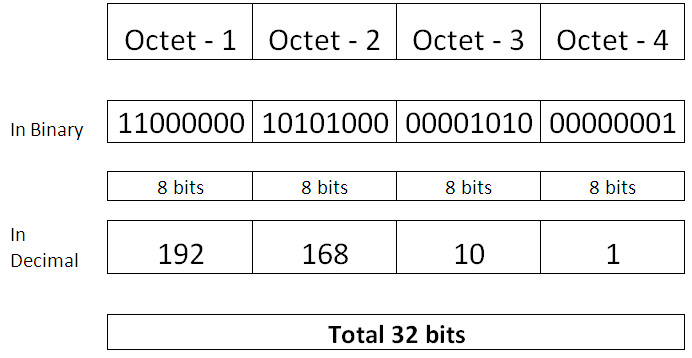If you convert the binary value to decimal for:

Octet -1 we get the equivalent 192

Octet -2 we get the equivalent 168

Octet -3 we get the equivalent 10

Octet -4 we get the equivalent 1

In the below table we see that after putting the Binary values and adding the values with 1’s, we get the decimal equivalent. E.g. 128 + 64 = 192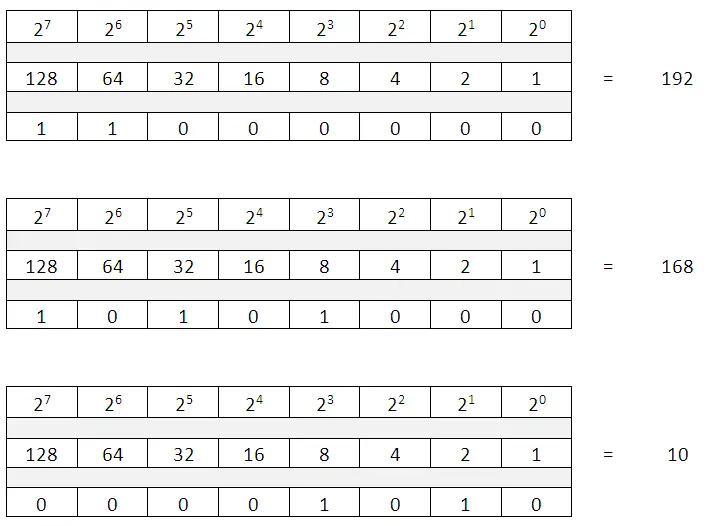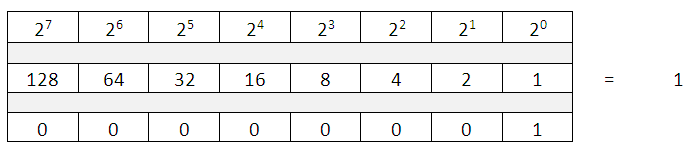The values in each octet can be of range 0 to 255 i.e. all bits in an octet set to 0 or 1.

IP addresses are classified into different classes, namely:

Class A    0 – 127

Class B   128 – 191

Class C   192 – 223

Class D  224 – 239

Class E   240 – 255

Classes A, B, C are the IP address which are used on the network i.e. on the LAN and WAN

Class D – IP address ranges are reserved for MULTICAST

Class E – IP address ranges is used for future purpose or research and development.

Based on the Class of IP address, we can determine the Network Portion and the Host Portion.

The Network specifies the set or group of devices where as the host means the end device or single device.

Class A, has first octet for Network and remaining 3 octets for Host  N. H. H. H

Class B, has first and second octet for Network and remaining 2 octets for Host  N. N. H. H

Class C, has first three octets for Network and remaining 1 octet for Host  N. N. N. H

So if we take the example of the IP address in Class C, i.e. 192.168.1.1

Seeing the first Octet value i.e. 192, we can make out this is a Class C, IP address.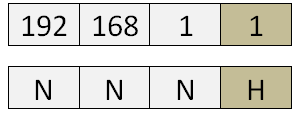In the above mentioned address as 192.168.1.x  ( x can be any number from 0 to 255) represent the network and the last octet can be any values from 0 to 255, all these IP address are part of the same network i.e. 192.168.1.0 network.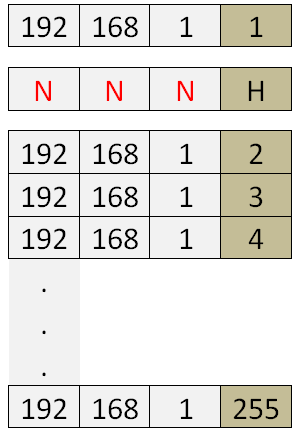A similar representation for Class B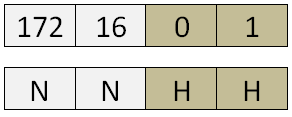A similar representation for Class A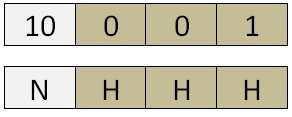Let’s see how many network and host can each class of IP address have.

Network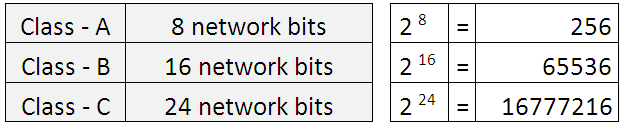Host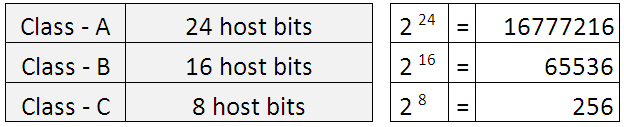Among all the IP address available for hosts not all are valid, we need to remove 2 from the available list as we cannot have the values of all 0’s and all 1’s used. The first and the last address are used for the network and Broadcast ID.

Thus the Valid number of host address becomes:

Host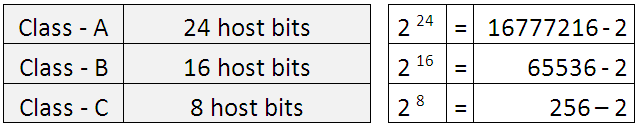Consider a Class C IP address range, 192.168.1.0, so the below picture gives an idea of the network address, valid IP addresses that can be used to assign to hosts and the broadcast address.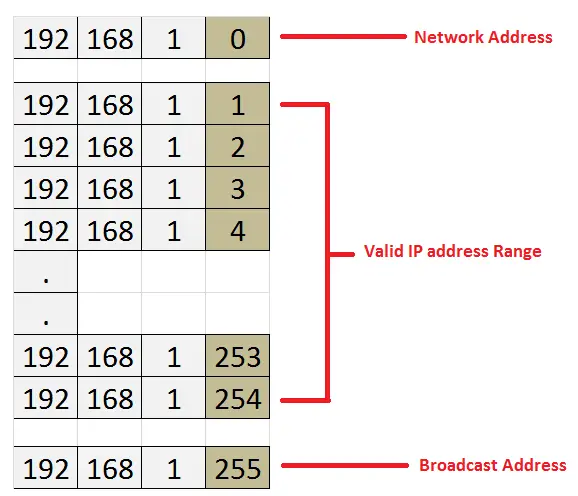The Network and Host portion are differentiated by the use of a Subnet Mask.  In a subnet Mask, all 1’s represent a Network and all 0’s represent hosts.

The default subnet masks for all the three Classes are as below.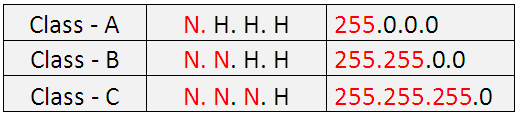Note: the IP address in range of 127.x.x.x is only used for testing and is commonly known as the loopback IP address.

There is a concept of PRIVATE IP Address, which means these IPs can be assigned to any device in a network and these IP address cannot communicate via the INTERNET (PUBLIC Network). The Private IP Addresses is discussed in detail in the RFC 1918. These addresses are reserved for Private networks and below are the range for each class.Subnetting

This is a very popular technique in IP addressing. It is used to divide a single Network into multiple small networks.

The concept of subnetting was introduced to reduce the wastage of IP addresses. For example: In a Class C network, we can have 254 valid IP address, means can connect up to 254 devices. But what if you only have 10 devices and you are using the Class C range, there are 244 IP address are wasted and cannot be used by someone else.

There are two ways subnetting can be done:

a)    FLSM – Fixed Length Subnet Mask

b)    VLSM – Variable Length Subnet Mask

We can do subnetting based on the requirement of Host and Networks.

Example, in a house while construction, you know the requirement that a drawing room needs to accommodate about 6 to 8 people, a bedroom should accommodate 2-3 people, thus you plan and design the house based on the it and decide the size. In Terms of networking and IP addressing, Hosts and Networks are the key for deciding requirements.

Hosts required?    2H >= requirement

Networks required? 2N >= requirement

Now let’s do an example of subnetting. Consider you have 4 departments, namely:

1.    Sales

2.    Accounts

3.    HR

4.    IT

Your manager wants you to design a network that each department has 50 hosts, i.e. in total 200 hosts.

With the above requirement you can very easily configure the class C IP address as it can have up to 254 valid hosts.

Thus if you use the IP address 192.168.1.0 network, you can accommodate all the 4 departments hosts and meet the requirements of your manager.

192.168.1.5 to 192.168.1.55  Sales

192.168.1.56 to 192.168.1.106  Accounts

192.168.1.107 to 192.168.1.157  HR

192.168.1.157 to 192.168.1.207  IT

The above scenario worked fine for some days, and you began to receive complaints that users from different departments are accessing data or other departments which they should not be doing i.e. Sales people are accessing data from HR department computers.

Now you need to redesign the IP addressing to make sure no such issues are reported. So you decide to give each department an IP address like below:So you have overcome the issue of departments access each other’s computers but at a cost of wasting 204 IP address in each network, and total wastage of about 204*4 = 816 IP addresses. This does not look like a good option. Thus we will see how subnetting can help on this.

We will make use of one block of 256 addresses and try and accommodate all the 4 departments in that. (Subnetting: process of dividing a default networks into further smaller networks to avoid wastage of IP addresses)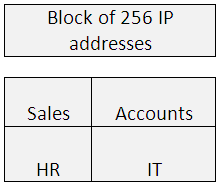FLSM:

If dividing the network into equal sizes, like of each network 64 addresses.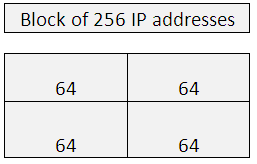VLSM:

If dividing the network into unequal sizes, like of one network of 128 addresses, other of 64, one of 32 and some further split into 16, 8, or 4 IP addresses.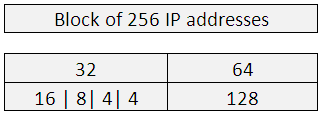When we do subnetting, what we are doing is converting some hosts bits to network bits. Or can say converting 0’s to 1’s.

Let me explain a little about slash notation ‘/’, you would have seen IP addresses mentioned like : 192.168.1.1/24, here the 24 represents the number of 1’s in the subnet mask.

192.168.1.1 With subnet mast of 255.255.255.0 can be written as 192.168.1.1/24

Please see below an important table that you should memorize to make subnetting easy for you.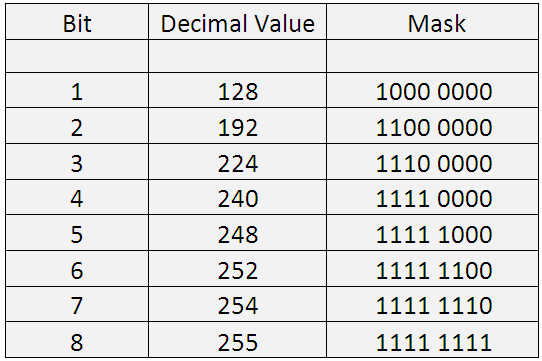One more example: if using 192.168.1.1 /29 means 29 bits marked as 1 in subnet mask. If you calculate the values by putting in the 29 times 1, you get.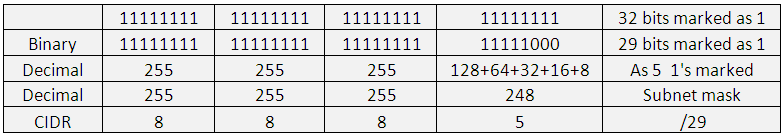You can see in the above table, As CIDR notation is /29, means 29  1’s, and the value in the last octet with 5  1’s equals to 248.

Thus: 192.168.1.1 with subnet mask of 255.255.255.248 can also be written as 192.168.1.1 /29.

Now proceeding with our requirement of 50 hosts while using network address 192.168.1.0 , the formula we use is:

Hosts required    2H >= requirement

So, 2^H >=50

Putting the value of H as mentioned below we get corresponding values.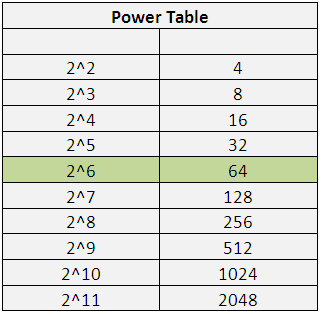Now, if we use 2 ^6, we get 64 hosts and, our requirement is 50 hosts. The formula holds true.

Hosts required    2^6 >= 50

Hosts required    64 >= 50 —– Condition is met, thus keep in mind the number ‘6’. We need 6 host bits to meet the requirement.

Out of the available 64 addresses, we cannot use the first and last IP address as they are reserved for Network address and broadcast address. Thus allowed usable IP addresses or valid host IPs is 64 -2 = 62. (Can connect up to 62 devices)

Note: You cannot divide the network to an exact value, but we try and divide it to the nearest possible value to avoid minimum wastage of IPs.

See the below table: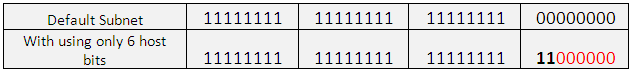The default host bits are 8, but to meet our requirement of 50 hosts, only 6 bits are required, thus the remaining two are provided to the network, and thus converted to 1.

So,

• Host bits required (h) =6
• Converted Network bits (n) = Total host bits – required host bits (h), 8 -6 =2
• Thus, converted Network bits (n) =2
• Total Network Bits =default Network Bits +  converted network Bits (n) = 24+2=26
• Hosts per Subnet = 2h -2 = 2 6 – 2 = 64 -2 = 62 hosts per subnet
• Subnets = 2 n = 2 2 = 4 ( As 2 bits were moved from host to subnet)
• Therefore customized subnet mask = /26 = 255.255.255.192
• Valid Range of IPs : = 2h -2 = 2 6 – 2 = 64 -2 = 62 hosts
• The networks will be in intervals of 64. That is: 0, 64, 128, and 192.

The networks will be in intervals of 64. That is: 0, 64, 128, and 192.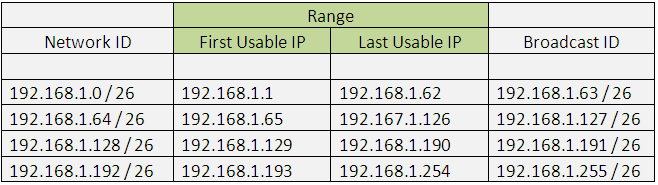VLSM:

Now assume the scenario where you need hosts as mentioned below:

Accounts: 100 hosts

Sales: 50 hosts

Marketing: 20 hosts

HR: 6 hosts

In this scenario, we need to use the VLSM concept. As in FLSM also we have wastage of IP addresses, thus VLSM can be used to divide to the nearest value.

In FLSM the network size is of equal size in VLSM network is of variable size.

We will use a Class C address, let’s start with the highest number of host required, i.e. 100. Using the formula we can derive the below.

2H -2 >= requirement

2 7 -2>=100

128 -2 >= 100

Required host bits: 7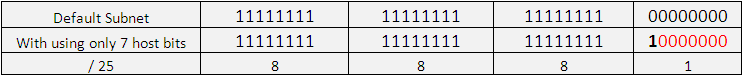Thus the subnet mask is: 255.255.255.128   or / 25

Department: Accounts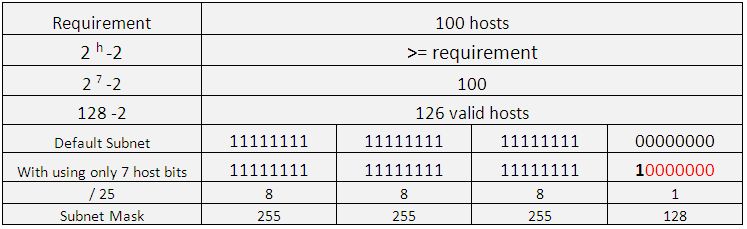Similar for the requirement of other departments:

Department: Sales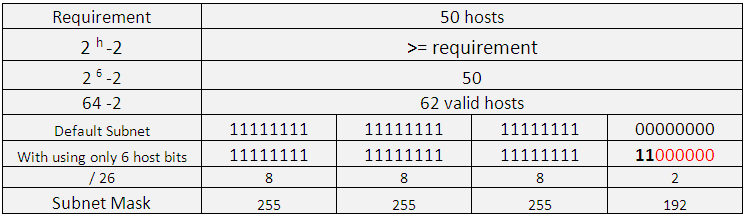Department: Marketing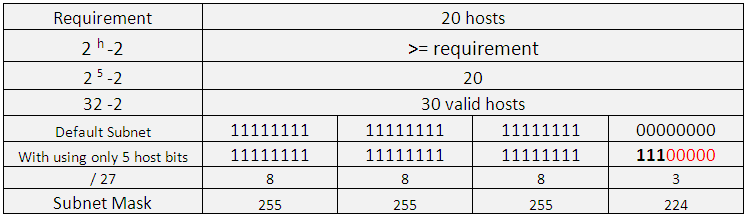Department: HR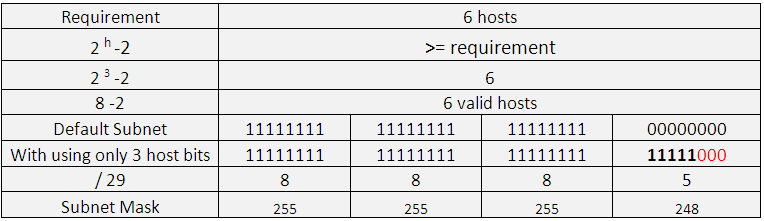Therefore the range would look like below: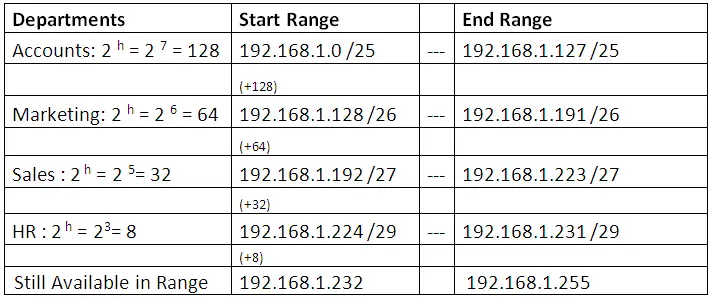Supernetting:

Supernetting is also known as aggregation or route summarization.

Taking a bunch of contiguous networks from your routing table and advertising these routes as a single route, that is summarized or aggregated is known as Route Summarization.

Benefits of summarization

A better routing environment is created as:

• The size of routing table is reduced which in turn takes less memory and processing
• As reducing size of routing update, the bandwidth required is less
• Avoids routing table update due to a flapping link

200.10.20.0/24 and 200.10.21.0 /24

Convert them to Binary

Perform the AND Operation.

Below is a table of how the results would appear when AND operation is performed.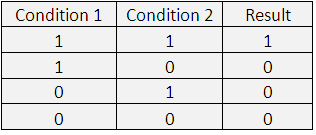Thus after ANDing, we see that from the default subnet mask of 24 bits,, 1 bit is given to the Host. Thus, only having 23 bits for Network.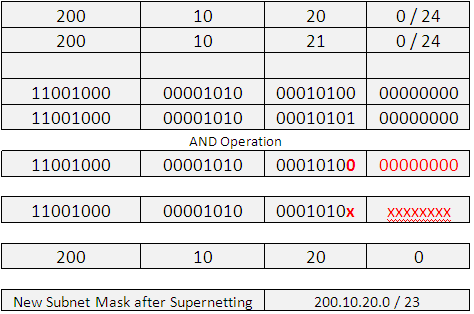Thus both the earlier networks 200.10.20.0/24 and 200.10.21.0 /24 can be written in as 200.10.20.0 / 23. Thus a router need not put both the entries in its routing table and can just keep one entry 200.10.20.0 / 23.

Consider one more example, with 4 Networks, also see the diagram.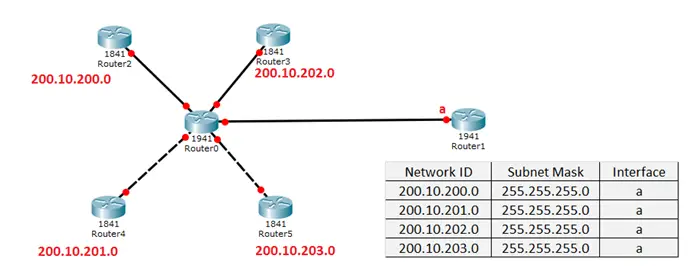In the above diagram, The Routing Table of Router1 has 4 entries for the networks connected to Router 0.

In an Ideal situation, if Router 1 send packet to Router 0, it should be the lookout of router 0 to forward the packets to the other connected routers. So if we summarize the Networks and provide that information to Router1, the entries in the routing table will reduce, thus have less Routing table size and less burden on the Router 1.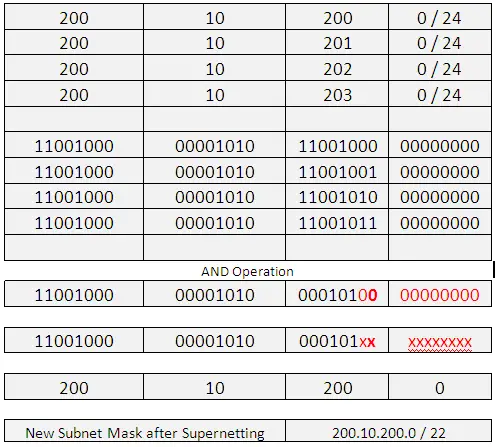In the above table we see the four IP addresses and their corresponding binary values.

Then the AND operation is performed and the resultant values are: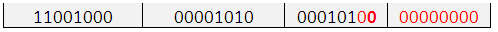If you convert these back to decimal you get: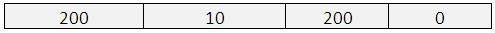And the subnet mask to be used with this is a / 22 as there are 22 network bits used.

So, all the four entries in a routing table can be replaced with 200.10.200.0 / 22.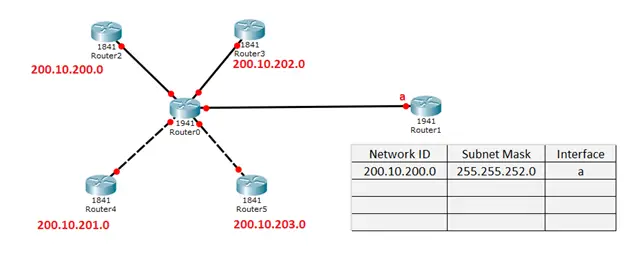As seen the routing table in the above diagram, the earlier 4 entries of routing table are replaced by only one entry. Thus, this reduces the overhead on the Router reduces entries in routing table.